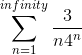# Sum of infinite series

## Homework Statement

Find the sum of the series## The Attempt at a Solution

Not sure exactly where to start. If I move 3 outside the sum I'm left with 3*sigma(1/n*4^n), which I can rewrite to 3*sigma((1/n)*(1/4)^n), which party looks like a geometric series..Any tips?

#### Attachments

•Delta2

RPinPA
Homework Helper
No, it's not a geometric series. The characteristic of a geometric series is that the ratio between successive terms is a constant. The ratio ##a_{n+1}/a_n## in this case is ##(n 4^n) / [(n+1)4^{n+1}]## or ##n/[4(n+1)]##, definitely not a constant.

That said, I'm not sure off-hand what trick might work here. Here's a PDF that shows some of the tricks for transforming infinite series into something that you can work with.
http://web.math.ucsb.edu/~cmart07/Evaluating Series.pdf

I think the basic hope here would be if you can use the Taylor series approach: Can you transform this into some known Taylor series, evaluated at a particular value? Then it would be that function at that value. Or perhaps the derivative of a known Taylor series? That's where I'd start looking, but I have no specific advice to give you.

Edit: I think I found one. Look at a table of common Taylor series and find one where the n-th term has an n in the denominator. You can make that work for you. That is, the n-th term of the expansion of ##f(x)## can be the same as the n-th term of this series, for a particular x.

•Delta2
Ray Vickson
Homework Helper
Dearly Missed

## Homework Statement

Find the sum of the series
View attachment 234061

## The Attempt at a Solution

Not sure exactly where to start. If I move 3 outside the sum I'm left with 3*sigma(1/n*4^n), which I can rewrite to 3*sigma((1/n)*(1/4)^n), which party looks like a geometric series..Any tips?

You need to figure out what is
$$F(x) = \sum_{n=1}^\infty \frac{x^n}{n}.$$ This is actually a well-known series that appears in all kinds of calculus textbooks and which you might have see already in your studies. Even if you do not recognize it, there are some standard tricks that people use when facing such series, such as looking at the series for ##dF(x)/dx## or ##\int F(x) \, dx##.

•Delta2
Delta2
Homework Helper
Gold Member
When you use integration you can use definite integration (instead of indefinite) and integrate from 0 to t which essentially will make the constant of integration zero.
In the left hand side you ll have

$$\int_0^t \sum (\frac{x^n}{n})'dx=\sum \frac{t^n}{n} -\sum \frac{0^n}{n}$$

and in the right hand side

$$\int_0^t\frac{1}{1-x}dx=-ln(1-t)+ln(1-0)$$

I use $$log(x)=\sum_{n=1}^{\infty}\frac{1}{n}\left (\frac {x-1}{x}\right )^n$$ for ## x>\frac {1}{2}## and set
##\frac {(x-1)}{x}=\frac {1}{4}## so ##x=\frac {4}{3}##